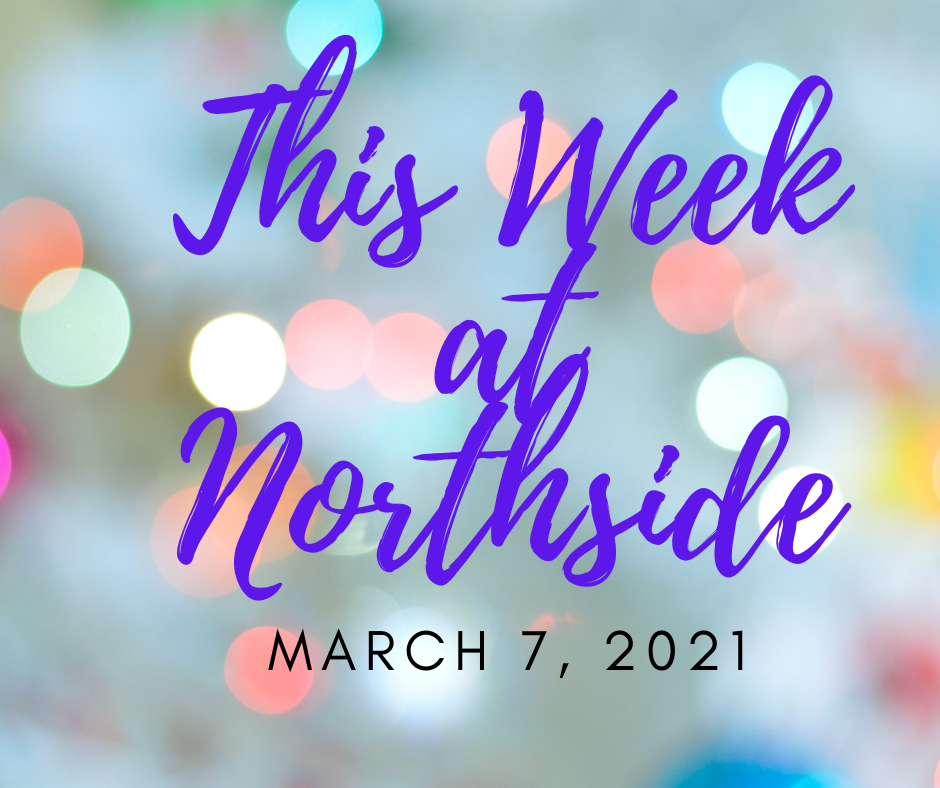/* styles */

# Upcoming Important Dates

Monday, March 8 - Deadline for Student Artwork for FON Spring Benefit
Tuesday, March 9 - Financial Aid Presentation for Juniors and Sophomores
Tuesday, March 16 - LSC Meeting
Tuesday, March 23 - Friends of Northside Meeting
Miércoles 31 de Marzo - Hora de Conversación en Español

This week's scheduled swim meets, badminton and basketball games, and bowling tournaments are listed in the Winter Sports article.

 table div table+table+table+table+table div table{width:100%;padding:0}table div table+table+table+table+table div table img{width:96.23%;padding:0;float:none}table div table+table+table+table+table div table td{width:100%;padding:0 1.88% 18px}/* styles */## News from the Counseling Office

▪ Financial Aid Presentation Planned for Juniors and Sophomores THIS Tuesday, March 9
▪ Update: Summer Enrichment/Pre-College Programs
▪ Chicago Teachers Union Scholarship
▪ Golden Apple Scholars of Illinois
▪ Military Academies Virtual Day Information Session
 ▪ Financial Aid Presentation Planned for Juniors and Sophomores THIS Tuesday, March 9
 ▪ Update: Summer Enrichment/Pre-College Programs
 ▪ Chicago Teachers Union Scholarship
 ▪ Golden Apple Scholars of Illinois
 ▪ Military Academies Virtual Day Information Session

## Sports, Yearbook & General School News

▪ LSC Vacancies
▪ Pandemic Electronic Benefit Program
▪ Future Problem Solvers Coaching Opportunity
▪ Winter Sports - Games This Week
▪ Upcoming Registration for Spring Sports: Deadlines for Girls Water Polo, Softball, Baseball, Girls Soccer, Boys & Girls Track & Field, Boys & Girls Lacross, Boys Tennis, Boys Volleyball
▪ Available Now: Custom NCP Brick With Your Personalized Message for Your Student
▪ Photo Submissions Requested for NCP Stampede!
▪ Yearbook Ads for Seniors Available
▪ Update on School Portraits for 9th, 10th, and 11th Graders
▪ Order Your 2020-2021 NCP Yearbook
▪ Ways for Parents to Stay in Touch/Volunteer/Support NCP/Social Media Links
 ▪ LSC Vacancies
 ▪ Pandemic Electronic Benefit Program
 ▪ Future Problem Solvers Coaching Opportunity
 ▪ Winter Sports - Games This Week
 ▪ Upcoming Registration for Spring Sports: Deadlines for Girls Water Polo, Softball, Baseball, Girls Soccer, Boys & Girls Track & Field, Boys & Girls Lacross, Boys Tennis, Boys Volleyball
 ▪ Available Now: Custom NCP Brick With Your Personalized Message for Your Student
 ▪ Photo Submissions Requested for NCP Stampede!
 ▪ Yearbook Ads for Seniors Available
 ▪ Update on School Portraits for 9th, 10th, and 11th Graders
 ▪ Order Your 2020-2021 NCP Yearbook
 ▪ Ways for Parents to Stay in Touch/Volunteer/Support NCP/Social Media Links
 table div table+table+table+table+table+table+table div table{width:100%;padding:0}table div table+table+table+table+table+table+table div table img{width:96.23%;padding:0;float:none}table div table+table+table+table+table+table+table div table td{width:100%;padding:0 1.88% 18px}/* styles */# FON Spring Benefit Call for Student Artwork: Deadline for Submissions Extended to Monday, March 8

Friends of Northside is looking for NCP students to help them visually illustrate their Spring Virtual Fundraising Event theme: "Together but Apart." The past year has been one like no other, and they would like to showcase the resilience, strength, and resourcefulness that our students, faculty, and parents have demonstrated and continue to make Northside a place that exemplifies what is possible in public education.

Students have been asked via NCP email to submit their creative interpretation of the event theme, “Together but Apart,” as a two-dimensional drawing, painting, computer illustration, collage, or other medium that can be scanned flat and sent as a high-resolution color 300dpi jpg, pdf, or eps file. Selected artwork will be featured in marketing materials and communications before and during the event.

The email contains a Google Form to submit their artwork. The deadline for submissions is now Monday, March 8.

 table div table+table+table+table+table+table+table+table+table+table div table{width:100%;padding:0}table div table+table+table+table+table+table+table+table+table+table div table img{width:96.23%;padding:0;float:none}table div table+table+table+table+table+table+table+table+table+table div table td{width:100%;padding:0 1.88% 18px}/* styles */# Financial Aid Presentation Planned for Juniors and Sophomores THIS Tuesday, March 9

Hello, Junior and Sophomore Parents,

On Tuesday, March 9, from 6:00 pm - 8:00 pm, the Northside College Prep Counseling Department will host a virtual financial aid presentation for students and parents and/or guardians.

This presentation is being offered to help your family prepare to navigate the financial aid process. During the presentation, the presenters will outline the financial aid process for the Free Application for Federal Student Aid (FAFSA), R.I.S.E. Application for undocumented and transgendered students, and need-based financial aid.

To ensure we are meeting the needs of the Northside Prep community, we are offering presentations in English and Spanish. Click here to register for the virtual financial aid presentation. You should have received a Google Meeting invitation by Friday, March 6.

If you have any questions or concerns, feel free to contact Ms. Moore at mcmoore6@cps.edu.

 table div table+table+table+table+table+table+table+table+table+table+table+table+table div table{width:100%;padding:0}table div table+table+table+table+table+table+table+table+table+table+table+table+table div table img{width:96.23%;padding:0;float:none}table div table+table+table+table+table+table+table+table+table+table+table+table+table div table td{width:100%;padding:0 1.88% 18px}/* styles */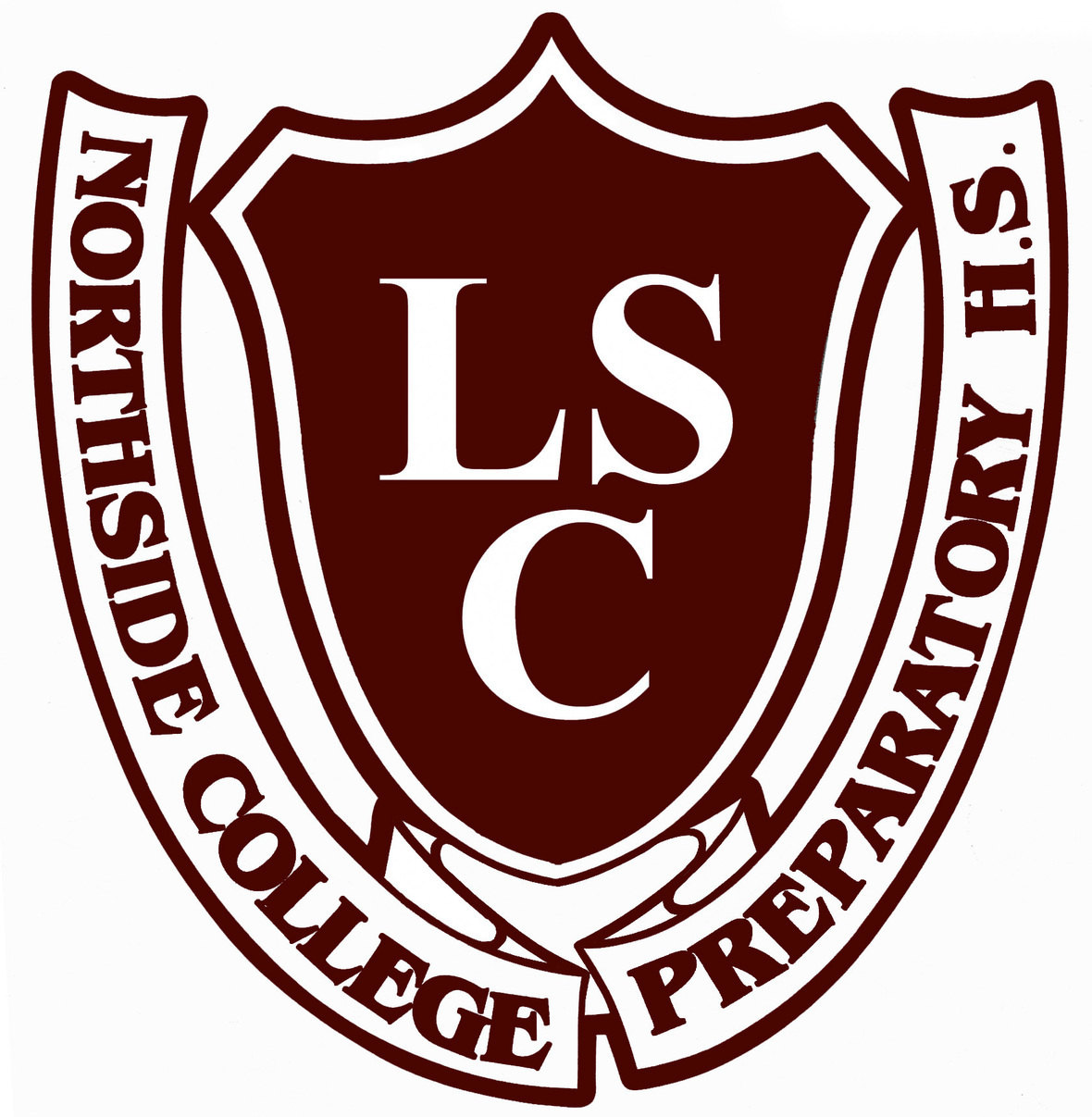# LSC Meeting

Tuesday, March 16
6:30 pm Zoom Meeting

 table div table+table+table+table+table+table+table+table+table+table+table+table+table+table+table div table{width:100%;padding:0}table div table+table+table+table+table+table+table+table+table+table+table+table+table+table+table div table img{width:96.23%;padding:0;float:none}table div table+table+table+table+table+table+table+table+table+table+table+table+table+table+table div table td{width:100%;padding:0 1.88% 18px}/* styles */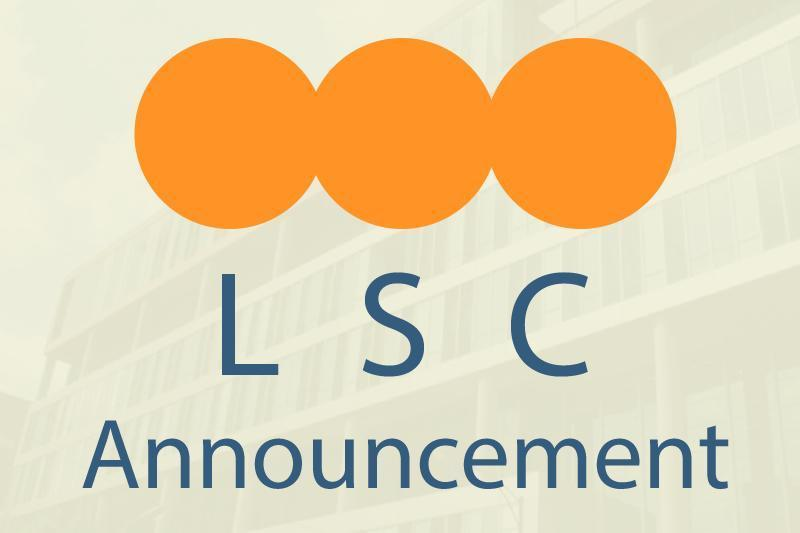# LSC Vacancies

The LSC has two vacancies: one for a parent and one for a community representative. All parents are encouraged to consider joining the LSC.

Please encourage active community members within the school boundaries to apply to be a community representative. They can see if they are within boundaries by entering their address HERE.

Applications must be submitted to school in person during school hours. Find the application forms HERE.

 table div table+table+table+table+table+table+table+table+table+table+table+table+table+table+table+table+table div table{width:100%;padding:0}table div table+table+table+table+table+table+table+table+table+table+table+table+table+table+table+table+table div table img{width:96.23%;padding:0;float:none}table div table+table+table+table+table+table+table+table+table+table+table+table+table+table+table+table+table div table td{width:100%;padding:0 1.88% 18px}/* styles */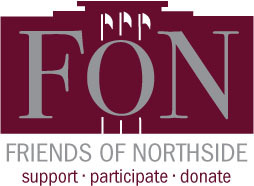# Friends of Northside

Tuesday, March 23

Please join Friends of Northside for their upcoming meeting.

Friends of Northside (FON) is the “one-stop-shop” for NCP families to get involved. FON facilitates communication between parents and the school, organizes parent volunteers, plans and sponsors social/educational events, and raises much-needed funds to bridge the gap due to CPS budget cuts. FON supports the school’s mission to maintain and enhance the learning experience for all students of Northside College Prep High School.

To find out more about Friends of Northside, please visit their website HERE.

 table div table+table+table+table+table+table+table+table+table+table+table+table+table+table+table+table+table+table+table div table{width:100%;padding:0}table div table+table+table+table+table+table+table+table+table+table+table+table+table+table+table+table+table+table+table div table img{width:96.23%;padding:0;float:none}table div table+table+table+table+table+table+table+table+table+table+table+table+table+table+table+table+table+table+table div table td{width:100%;padding:0 1.88% 18px}/* styles */# Hora de Conversación en Español

Miércoles 31 de Marzo
7:00 pm

Se llevará a cabo el miércoles 31 de marzo a las 7:00 pm.

 table div table+table+table+table+table+table+table+table+table+table+table+table+table+table+table+table+table+table+table+table+table div table{width:100%;padding:0}table div table+table+table+table+table+table+table+table+table+table+table+table+table+table+table+table+table+table+table+table+table div table img{width:96.23%;padding:0;float:none}table div table+table+table+table+table+table+table+table+table+table+table+table+table+table+table+table+table+table+table+table+table div table td{width:100%;padding:0 1.88% 18px}/* styles */# Pandemic Electronic Benefit Program

All CPS students, regardless of income or citizenship, are eligible to receive food benefits as part of the US Government’s COVID-19 pandemic response. Funds will be loaded onto cards that will be mailed to each student at the primary address listed for them in ASPEN. To make sure your student receives the benefits check that your primary address is up to date in ASPEN.

Watch the mail for a plain envelope due to arrive this month. Be sure to keep the card because additional benefits will be loaded onto it going forward. For more information, check the March 3 post on SchoolCNXT.

 table div table+table+table+table+table+table+table+table+table+table+table+table+table+table+table+table+table+table+table+table+table+table+table div table{width:100%;padding:0}table div table+table+table+table+table+table+table+table+table+table+table+table+table+table+table+table+table+table+table+table+table+table+table div table img{width:96.23%;padding:0;float:none}table div table+table+table+table+table+table+table+table+table+table+table+table+table+table+table+table+table+table+table+table+table+table+table div table td{width:100%;padding:0 1.88% 18px}/* styles */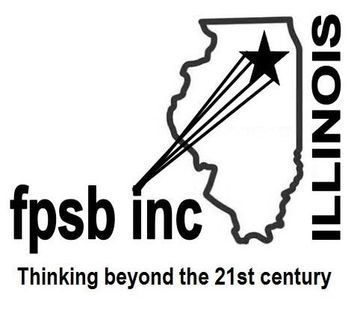# Future Problem Solvers Coaching Opportunity

The Future Problem Solvers Club needs a new coach for next year. FPS teams use the Six-Step Process to create an action plan to solve a problem identified in a scenario of a future situation. The teams of up to four students can compete in regional, state, and international level competitions.

Northside has typically fielded at least one team per grade. Individual competitions are also available. Whether students are interested in the arts and humanities or in STEM subjects, the Six-Step Process allows them to use their strengths in collaboration with other students as they identify challenges and articulate underlying problems, brainstorm possible solutions, generate criteria to evaluate those solutions, and then come up with an action plan to address the problem.

Training is required and provided by FPS Illinois. For more information, contact Janice Cody at jcody6933@gmail.com.

 table div table+table+table+table+table+table+table+table+table+table+table+table+table+table+table+table+table+table+table+table+table+table+table+table+table div table{width:100%;padding:0}table div table+table+table+table+table+table+table+table+table+table+table+table+table+table+table+table+table+table+table+table+table+table+table+table+table div table img{width:96.23%;padding:0;float:none}table div table+table+table+table+table+table+table+table+table+table+table+table+table+table+table+table+table+table+table+table+table+table+table+table+table div table td{width:100%;padding:0 1.88% 18px}/* styles */# Update: Summer Enrichment/Pre-College Programs

In addition to the virtual information sessions Northside Prep is offering after school daily and during the school day on Wednesdays (click HERE), the counseling department would like to share additional information about information sessions for summer enrichment opportunities.

Are you and your student interested in learning about enrichment opportunities or pre-college programs to engage in during the spring or summer? Would you like to build your resume and enhance your academic, personal, or social skills? If the answer is yes, consider reviewing the following resources to assist your family with identifying the best fit enrichment opportunities for your student:

 table div table+table+table+table+table+table+table+table+table+table+table+table+table+table+table+table+table+table+table+table+table+table+table+table+table+table+table+table div table{width:100%;padding:0}table div table+table+table+table+table+table+table+table+table+table+table+table+table+table+table+table+table+table+table+table+table+table+table+table+table+table+table+table div table img{width:96.23%;padding:0;float:none}table div table+table+table+table+table+table+table+table+table+table+table+table+table+table+table+table+table+table+table+table+table+table+table+table+table+table+table+table div table td{width:100%;padding:0 1.88% 18px}/* styles */# Chicago Teachers Union Scholarship

The Chicago Teachers Union Scholarship Committee is working hard to adapt and maintain our annual tradition of awarding scholarships to children of our members who are graduating and have plans to pursue post-secondary education. If you have a child who will be graduating from high school this year, NOW is the time for them to apply for a CTU/CTUF Scholarship! The deadline is May 10, 2021.

Scholarships in the amount of \$1,000 will be awarded to students to help defray the cost of post-secondary education in college or vocational programs. Details are available at www.ctulocal1.org/scholarship.

## Eligibility

Awards are entirely unrestricted as to race, religion, political affiliation, or choice of an accredited college, university, business, trade, or vocational school.

The applicant must be a child of a currently employed, active member in good standing of the Chicago Teachers Union, or a child of a deceased member who was in good standing with the Chicago Teachers Union at the time of death. They must also be a high school senior who will graduate this year before August 31, 2020. Special education students are eligible for all scholarships and are encouraged to apply.

## How to Apply

Please make certain that the application is completed in full, and that it is signed by the applicant AND the parent or guardian so that membership in good standing can be verified before presentation to the Scholarship Committee of the Chicago Teachers Union.

## Application Info

Scholarship winners will be notified by mail or email and invited to attend a special virtual reception and award ceremony with their families.

 table div table+table+table+table+table+table+table+table+table+table+table+table+table+table+table+table+table+table+table+table+table+table+table+table+table+table+table+table+table+table div table{width:100%;padding:0}table div table+table+table+table+table+table+table+table+table+table+table+table+table+table+table+table+table+table+table+table+table+table+table+table+table+table+table+table+table+table div table img{width:96.23%;padding:0;float:none}table div table+table+table+table+table+table+table+table+table+table+table+table+table+table+table+table+table+table+table+table+table+table+table+table+table+table+table+table+table+table div table td{width:100%;padding:0 1.88% 18px}/* styles */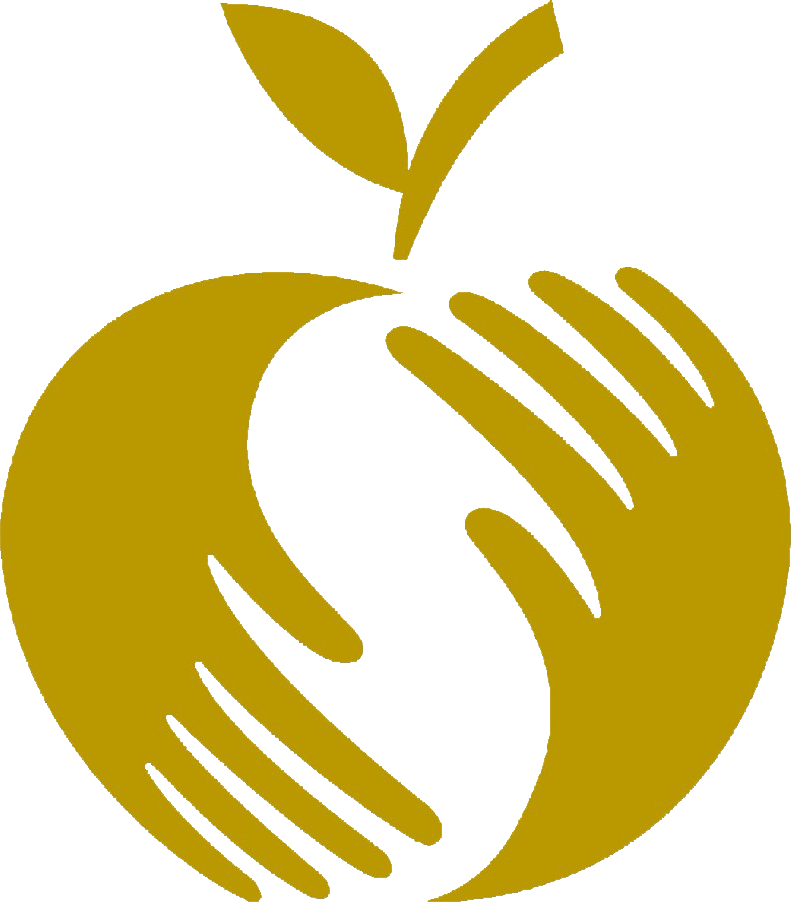# Golden Apple Scholars of Illinois

Do you know students in your school or district who would be great teachers? The Golden Apple Scholars teacher preparation and tuition assistance program is accepting applications for the Class of 2021 until April 1!

The Scholars recruitment team will be hosting live information sessions where interested students can learn more about the program, application process, and benefits.

Sessions will be held weekly at 6:00 pm on the following dates:

▪ Tuesday, March 2
▪ Tuesday, March 9
▪ Tuesday, March 16
▪ Tuesday, March 23
▪ Tuesday, March 30

Students can register HERE and we would greatly appreciate your support in sharing this information with students at your school.

▪ Up to \$23,000 in financial assistance for college, inclusive of paid Scholar Institutes
▪ Extensive classroom teaching experience
▪ Job placement assistance
▪ Mentoring from our award-winning teaching faculty

## Who Is Eligible?

▪ Students attending Illinois schools who are high school seniors, freshmen/sophomores in college and community college students
▪ Illinois resident, U.S. citizens, eligible non-citizens, or Illinois Deferred Action for Childhood Arrivals (DACA) students

 table div table+table+table+table+table+table+table+table+table+table+table+table+table+table+table+table+table+table+table+table+table+table+table+table+table+table+table+table+table+table+table+table div table{width:100%;padding:0}table div table+table+table+table+table+table+table+table+table+table+table+table+table+table+table+table+table+table+table+table+table+table+table+table+table+table+table+table+table+table+table+table div table img{width:96.23%;padding:0;float:none}table div table+table+table+table+table+table+table+table+table+table+table+table+table+table+table+table+table+table+table+table+table+table+table+table+table+table+table+table+table+table+table+table div table td{width:100%;padding:0 1.88% 18px}/* styles */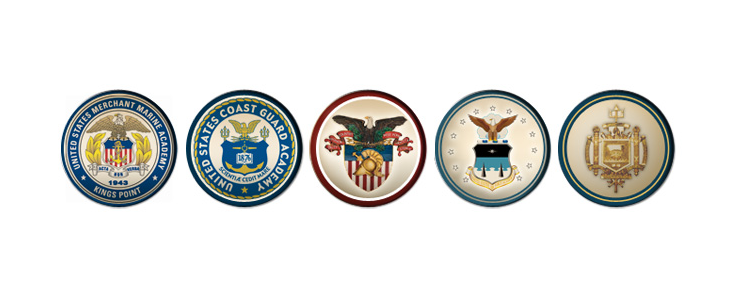# Military Academies Virtual Day Information Session

If you would like to learn how to earn a college degree, serve your country, and graduate with a high-paying career and no college debt, join us for Academy Day on March 20, 2021,, sponsored by Senators Durbin and Duckworth.

Representatives for each service Academy (United States Military Academy, United States Air Force Academy, United States Naval Academy, United States Coast Guard Academy, United States Merchant Marine Academy) and ROTC (Reserve Officers’ Training Corps) will share how each program prepares the future leaders for our military. They will also provide resources to help you with the application process.

Register HERE for the event. We will start with overviews of each program followed by breakouts and an opportunity for you to ask questions from admissions representatives.

A link to the zoom event will be sent to you by March 18 at the email you use to register.

Best of luck,

Kenzo Murray
United States Military Academy at West Point Field Force Member

 table div table+table+table+table+table+table+table+table+table+table+table+table+table+table+table+table+table+table+table+table+table+table+table+table+table+table+table+table+table+table+table+table+table+table div table{width:100%;padding:0}table div table+table+table+table+table+table+table+table+table+table+table+table+table+table+table+table+table+table+table+table+table+table+table+table+table+table+table+table+table+table+table+table+table+table div table img{width:96.23%;padding:0;float:none}table div table+table+table+table+table+table+table+table+table+table+table+table+table+table+table+table+table+table+table+table+table+table+table+table+table+table+table+table+table+table+table+table+table+table div table td{width:100%;padding:0 1.88% 18px}/* styles */# Winter Sports - Games This Week

Boys Swimming
▪ Monday, March 8 vs. Jones (away) @ 5:00 pm
▪ Tuesday, March 9 vs. Taft @ NCP at 5:00 pm
▪ Thursday, March 11 vs. Lane Tech @ NCP at 5:00 pm

▪ Monday, March 8 vs. Mather @ NCP at 5:30 pm
▪ Friday, March 12 vs. Payton @ NCP at 5:00 pm

▪ Wednesday, March 10 vs. Prosser @ NCP (Soph. at 5:00 pm; V at 7:00 pm)
▪ Friday, March 12 vs. Von Steuben (away) (Soph. at 5:00 pm; V at 7:00 pm)
▪ Saturday, March 13 vs. vs. Lakeview (away) (Freshman at 9:00 am)
▪ Saturday, March 13 vs. Foreman (away) (Soph. at 11:30 am; V at 2:00 pm)

▪ Tuesday, March 9 vs. Westinghouse (away) (JV at 5:00 pm; V at 7:00 pm)
▪ Thursday, March 11 vs. Payton @ NCP (JV at 5:00 pm; V at 7:00 pm)
▪ Saturday, March 13 vs. DePaul Prep @ DPP (Varsity only at 10 am)

Boys and Girls Bowling
▪ Tuesday, March 9 vs. Payton @ Fireside Bowl (5:00 pm)

## Videographers Needed

Unfortunately, under CPS and Illinois safety protocols for indoor sports, there are no spectators allowed in the gyms this season. But in true Mustang spirit, the home opener games for both Girls Basketball teams were live-streamed for parents to view online. A huge thanks to parents Neal Wilson and Rick Petrillo for making that happen.

AD Henderson has indicated that he is open to having parent volunteers record or live-stream sporting events in this non-traditional season (one per game for safety reasons). If any parents would like to volunteer to film any home games, matches, or meets on their iPad or video camera, please let Mr. Henderson know in advance by contacting him at hhenderson@cps.edu, or get in touch with Mike Hughes at mhughes@nge.com to coordinate. It’s a great way to keep other parents, students, and fans connected to the school community, from the comfort of home... where the popcorn is free!

 table div table+table+table+table+table+table+table+table+table+table+table+table+table+table+table+table+table+table+table+table+table+table+table+table+table+table+table+table+table+table+table+table+table+table+table+table div table{width:100%;padding:0}table div table+table+table+table+table+table+table+table+table+table+table+table+table+table+table+table+table+table+table+table+table+table+table+table+table+table+table+table+table+table+table+table+table+table+table+table div table img{width:96.23%;padding:0;float:none}table div table+table+table+table+table+table+table+table+table+table+table+table+table+table+table+table+table+table+table+table+table+table+table+table+table+table+table+table+table+table+table+table+table+table+table+table div table td{width:100%;padding:0 1.88% 18px}/* styles */# Upcoming Registration for Spring Sports

## Deadlines for Girls Water Polo, Softball, Baseball, Girls Soccer, Boys & Girls Track & Field, Boys & Girls Lacross, Boys Tennis, Boys Volleyball

Just a reminder that on-line registration for girls' water polo will end on Thursday, March 11.

Registration for softball, baseball, girls soccer, boys & girls track and field, boys & girls lacrosse, boys tennis, and boys volleyball will end on March 22.

Parents can register their students on the NCP athletic website by clicking on the activity registration tab at the top of the page. All students must register and must have a current physical on file in order to try out for a sport at NCP.

The athletic department will publish tryout and practice information as it becomes available. Be sure to look for a message via SchoolCNXT and check the NCP athletic website HERE for any updates.

 table div table+table+table+table+table+table+table+table+table+table+table+table+table+table+table+table+table+table+table+table+table+table+table+table+table+table+table+table+table+table+table+table+table+table+table+table+table+table div table{width:100%;padding:0}table div table+table+table+table+table+table+table+table+table+table+table+table+table+table+table+table+table+table+table+table+table+table+table+table+table+table+table+table+table+table+table+table+table+table+table+table+table+table div table img{width:96.23%;padding:0;float:none}table div table+table+table+table+table+table+table+table+table+table+table+table+table+table+table+table+table+table+table+table+table+table+table+table+table+table+table+table+table+table+table+table+table+table+table+table+table+table div table td{width:100%;padding:0 1.88% 18px}/* styles */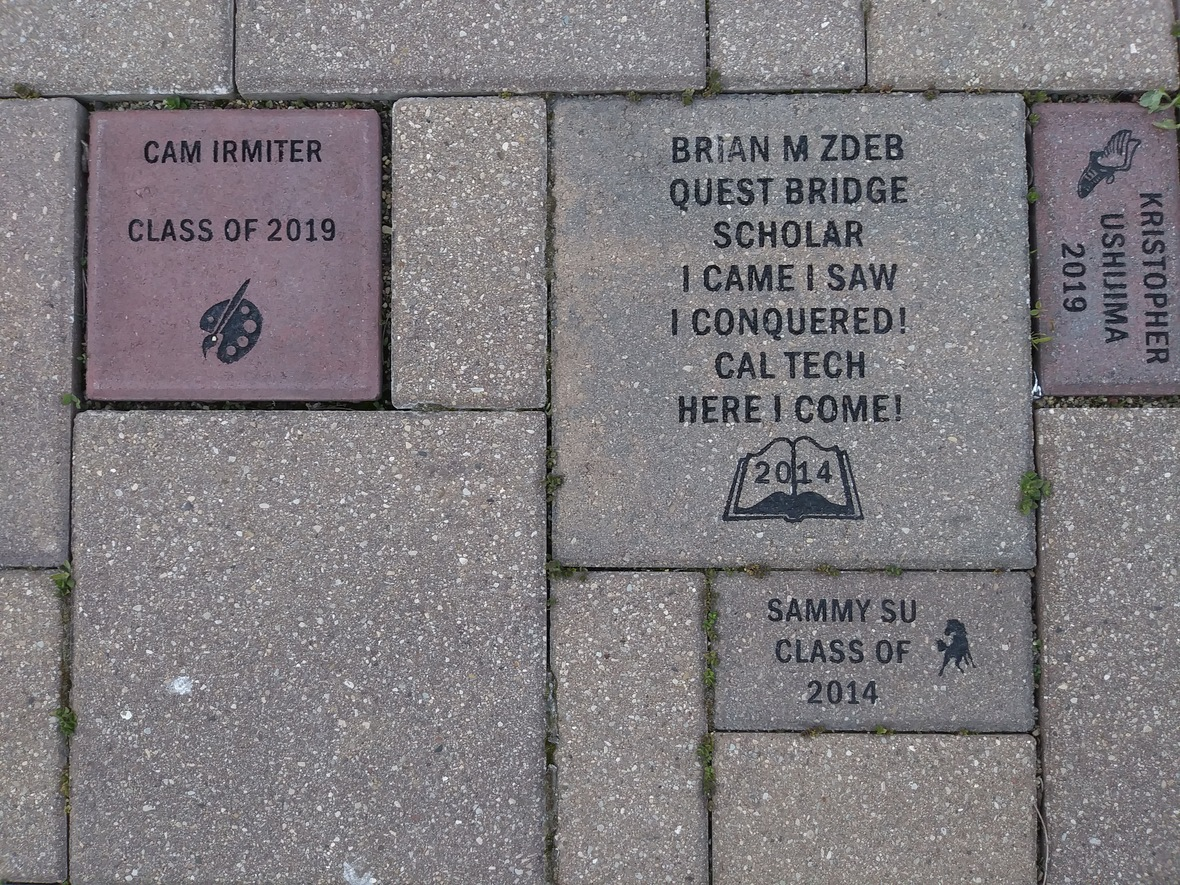Consider buying a custom NCP Brick for your child this year. Your brick, engraved with a personal message, will become a permanent part of the Northside College Prep Celestial Garden Walkway.

Your brick may be purchased in three sizes:

▪ 4" x 8" = \$50
▪ 8" x 8" = \$100
▪ 12" x 12" = \$150

Friends of Northside will provide a gift certificate acknowledging the purchase. Janet Jasmer will assist with the process and can answer any questions at jjasmer@atproperties.com.

 table div table+table+table+table+table+table+table+table+table+table+table+table+table+table+table+table+table+table+table+table+table+table+table+table+table+table+table+table+table+table+table+table+table+table+table+table+table+table+table+table+table div table{width:100%;padding:0}table div table+table+table+table+table+table+table+table+table+table+table+table+table+table+table+table+table+table+table+table+table+table+table+table+table+table+table+table+table+table+table+table+table+table+table+table+table+table+table+table+table div table img{width:96.23%;padding:0;float:none}table div table+table+table+table+table+table+table+table+table+table+table+table+table+table+table+table+table+table+table+table+table+table+table+table+table+table+table+table+table+table+table+table+table+table+table+table+table+table+table+table+table div table td{width:100%;padding:0 1.88% 18px}/* styles */# Any Questions About NCP Communications Sent Via CNXT?

If you are having problems with SchoolCNXT, please contact the main office from 7:30 am - 3:15 pm at 773-534-3954, or you can email the NCP school clerk, Mary Grasz, at mtgrasz@cpu.edu.

NCP uses SchoolCNXT to communicate important information to parents and the school community. All families should have received a text message and/or email communication about signing up. If you did not receive that communication or need access to your student's account, you can gain access by using this tool on Northside's website.

 table div table+table+table+table+table+table+table+table+table+table+table+table+table+table+table+table+table+table+table+table+table+table+table+table+table+table+table+table+table+table+table+table+table+table+table+table+table+table+table+table+table+table+table+table div table{width:100%;padding:0}table div table+table+table+table+table+table+table+table+table+table+table+table+table+table+table+table+table+table+table+table+table+table+table+table+table+table+table+table+table+table+table+table+table+table+table+table+table+table+table+table+table+table+table+table div table img{width:96.23%;padding:0;float:none}table div table+table+table+table+table+table+table+table+table+table+table+table+table+table+table+table+table+table+table+table+table+table+table+table+table+table+table+table+table+table+table+table+table+table+table+table+table+table+table+table+table+table+table+table div table td{width:100%;padding:0 1.88% 18px}/* styles */table.module-44{width:61.89%;padding:0}table div table+table+table+table+table+table+table+table+table+table+table+table+table+table+table+table+table+table+table+table+table+table+table+table+table+table+table+table+table+table+table+table+table+table+table+table+table+table+table+table+table+table+table+table+table div table{width:61.89%;float:none;margin-left:auto;margin-right:auto;padding:0}table div table+table+table+table+table+table+table+table+table+table+table+table+table+table+table+table+table+table+table+table+table+table+table+table+table+table+table+table+table+table+table+table+table+table+table+table+table+table+table+table+table+table+table+table+table div table a{border:0 none;text-decoration:none}table div table+table+table+table+table+table+table+table+table+table+table+table+table+table+table+table+table+table+table+table+table+table+table+table+table+table+table+table+table+table+table+table+table+table+table+table+table+table+table+table+table+table+table+table+table div table img{width:100%!important;border:0 none;text-decoration:none}table div table+table+table+table+table+table+table+table+table+table+table+table+table+table+table+table+table+table+table+table+table+table+table+table+table+table+table+table+table+table+table+table+table+table+table+table+table+table+table+table+table+table+table+table+table div table td{width:100%;padding:0}/* styles */

# 2020-2021 Yearbook Ads for Seniors Now Available

Seniors now have the opportunity to place ads in the 2020-2021 yearbook by visiting the yearbook website. After clicking on "continue", click on "start your ad" to begin.

# School Portraits for 9th, 10th, and 11th Graders

This letter was sent in CNXT explaining the process for submitting your student's school portrait to be included in the yearbook. The NCP code is 21740 if needed. If you have any questions, contact Ms. McCreedy at mkmccreedy@cps.edu.

 table.module-47{width:68.87%;padding:0}table div table+table+table+table+table+table+table+table+table+table+table+table+table+table+table+table+table+table+table+table+table+table+table+table+table+table+table+table+table+table+table+table+table+table+table+table+table+table+table+table+table+table+table+table+table+table+table+table div table{width:68.87%;float:none;margin-left:auto;margin-right:auto;padding:0}table div table+table+table+table+table+table+table+table+table+table+table+table+table+table+table+table+table+table+table+table+table+table+table+table+table+table+table+table+table+table+table+table+table+table+table+table+table+table+table+table+table+table+table+table+table+table+table+table div table a{border:0 none;text-decoration:none}table div table+table+table+table+table+table+table+table+table+table+table+table+table+table+table+table+table+table+table+table+table+table+table+table+table+table+table+table+table+table+table+table+table+table+table+table+table+table+table+table+table+table+table+table+table+table+table+table div table img{width:100%!important;border:0 none;text-decoration:none}table div table+table+table+table+table+table+table+table+table+table+table+table+table+table+table+table+table+table+table+table+table+table+table+table+table+table+table+table+table+table+table+table+table+table+table+table+table+table+table+table+table+table+table+table+table+table+table+table div table td{width:100%;padding:0}/* styles */

# Order a Yearbook Today!

Interested in purchasing a copy of the 2020-2021 Stampede, NCP's yearbook? Click HERE to start your order! And if you didn't get a chance to purchase a copy of the 2019-2020 yearbook, you now have a change to buy one of those, too! Just email Ms. McCreedy at mkmccreedy@cps.edu after you've completed your 2020-2021 order.

Copies are now \$55.

 table div table+table+table+table+table+table+table+table+table+table+table+table+table+table+table+table+table+table+table+table+table+table+table+table+table+table+table+table+table+table+table+table+table+table+table+table+table+table+table+table+table+table+table+table+table+table+table+table+table+table+table div table{width:100%;padding:0}table div table+table+table+table+table+table+table+table+table+table+table+table+table+table+table+table+table+table+table+table+table+table+table+table+table+table+table+table+table+table+table+table+table+table+table+table+table+table+table+table+table+table+table+table+table+table+table+table+table+table+table div table img{width:96.23%;padding:0;float:none}table div table+table+table+table+table+table+table+table+table+table+table+table+table+table+table+table+table+table+table+table+table+table+table+table+table+table+table+table+table+table+table+table+table+table+table+table+table+table+table+table+table+table+table+table+table+table+table+table+table+table+table div table td{width:100%;padding:0 1.88% 18px}/* styles */# Do you have news or team results to share with the Northside parent community?

 table div table+table+table+table+table+table+table+table+table+table+table+table+table+table+table+table+table+table+table+table+table+table+table+table+table+table+table+table+table+table+table+table+table+table+table+table+table+table+table+table+table+table+table+table+table+table+table+table+table+table+table+table+table+table div table{width:100%;padding:0}table div table+table+table+table+table+table+table+table+table+table+table+table+table+table+table+table+table+table+table+table+table+table+table+table+table+table+table+table+table+table+table+table+table+table+table+table+table+table+table+table+table+table+table+table+table+table+table+table+table+table+table+table+table+table div table img{width:96.23%;padding:0;float:none}table div table+table+table+table+table+table+table+table+table+table+table+table+table+table+table+table+table+table+table+table+table+table+table+table+table+table+table+table+table+table+table+table+table+table+table+table+table+table+table+table+table+table+table+table+table+table+table+table+table+table+table+table+table+table div table td{width:100%;padding:0 1.88% 18px}/* styles */# Support Northside - Shop AmazonSmile

AmazonSmile is a simple and automatic way to support Northside every time you shop.

Please note that our AmazonSmile name is Friends of "North" "Side" (North Side - two words at AmazonSmile).

 table div table+table+table+table+table+table+table+table+table+table+table+table+table+table+table+table+table+table+table+table+table+table+table+table+table+table+table+table+table+table+table+table+table+table+table+table+table+table+table+table+table+table+table+table+table+table+table+table+table+table+table+table+table+table+table+table div table{width:100%;padding:0}table div table+table+table+table+table+table+table+table+table+table+table+table+table+table+table+table+table+table+table+table+table+table+table+table+table+table+table+table+table+table+table+table+table+table+table+table+table+table+table+table+table+table+table+table+table+table+table+table+table+table+table+table+table+table+table+table div table img{width:96.23%;padding:0;float:none}table div table+table+table+table+table+table+table+table+table+table+table+table+table+table+table+table+table+table+table+table+table+table+table+table+table+table+table+table+table+table+table+table+table+table+table+table+table+table+table+table+table+table+table+table+table+table+table+table+table+table+table+table+table+table+table+table div table td{width:100%;padding:0 1.88% 18px}/* styles */# Follow Us on Social Media

 table div table+table+table+table+table+table+table+table+table+table+table+table+table+table+table+table+table+table+table+table+table+table+table+table+table+table+table+table+table+table+table+table+table+table+table+table+table+table+table+table+table+table+table+table+table+table+table+table+table+table+table+table+table+table+table+table+table+table div table{width:100%;padding:0}table div table+table+table+table+table+table+table+table+table+table+table+table+table+table+table+table+table+table+table+table+table+table+table+table+table+table+table+table+table+table+table+table+table+table+table+table+table+table+table+table+table+table+table+table+table+table+table+table+table+table+table+table+table+table+table+table+table+table div table img{width:96.23%;padding:0;float:none}table div table+table+table+table+table+table+table+table+table+table+table+table+table+table+table+table+table+table+table+table+table+table+table+table+table+table+table+table+table+table+table+table+table+table+table+table+table+table+table+table+table+table+table+table+table+table+table+table+table+table+table+table+table+table+table+table+table+table div table td{width:100%;padding:0 1.88% 18px}/* styles */table div table+table+table+table+table+table+table+table+table+table+table+table+table+table+table+table+table+table+table+table+table+table+table+table+table+table+table+table+table+table+table+table+table+table+table+table+table+table+table+table+table+table+table+table+table+table+table+table+table+table+table+table+table+table+table+table+table+table+table+table div table{width:100%;padding:0}table div table+table+table+table+table+table+table+table+table+table+table+table+table+table+table+table+table+table+table+table+table+table+table+table+table+table+table+table+table+table+table+table+table+table+table+table+table+table+table+table+table+table+table+table+table+table+table+table+table+table+table+table+table+table+table+table+table+table+table+table div table img{width:96.23%;padding:0;float:none}table div table+table+table+table+table+table+table+table+table+table+table+table+table+table+table+table+table+table+table+table+table+table+table+table+table+table+table+table+table+table+table+table+table+table+table+table+table+table+table+table+table+table+table+table+table+table+table+table+table+table+table+table+table+table+table+table+table+table+table+table div table td{width:100%;padding:0 1.88% 18px}/* styles */table div table+table+table+table+table+table+table+table+table+table+table+table+table+table+table+table+table+table+table+table+table+table+table+table+table+table+table+table+table+table+table+table+table+table+table+table+table+table+table+table+table+table+table+table+table+table+table+table+table+table+table+table+table+table+table+table+table+table+table+table+table+table div table{width:100%;padding:0}table div table+table+table+table+table+table+table+table+table+table+table+table+table+table+table+table+table+table+table+table+table+table+table+table+table+table+table+table+table+table+table+table+table+table+table+table+table+table+table+table+table+table+table+table+table+table+table+table+table+table+table+table+table+table+table+table+table+table+table+table+table+table div table img{width:96.23%;padding:0;float:none}table div table+table+table+table+table+table+table+table+table+table+table+table+table+table+table+table+table+table+table+table+table+table+table+table+table+table+table+table+table+table+table+table+table+table+table+table+table+table+table+table+table+table+table+table+table+table+table+table+table+table+table+table+table+table+table+table+table+table+table+table+table+table div table td{width:100%;padding:0 1.88% 18px}/* styles */